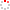CIOMP OpenIR  > 中科院长春光机所知识产出
 Parameter estimation in exponential models by linear and nonlinear fitting methods Yang, P.; C. P. Wu; Y. L. Guo; H. B. Liu; H. Huang; H. Z. Wang; S. Y. Zhan; B. Y. Tao; Q. Q. Mu; Q. Wang and H. Song 2017 发表期刊 Frontiers of Information Technology & Electronic Engineering卷号 18期号:3 摘要 Estimation of unknown parameters in exponential models by linear and nonlinear fitting methods is discussed. Based on the extreme value theorem and Taylor series expansion, it is proved theoretically that the parameters estimated by the linear fitting method alone cannot minimize the sum of the squared residual errors in the measurement data when measurement noise is involved in the data. Numerical simulation is performed to compare the performance of the linear and nonlinear fitting methods. Simulation results show that the linear method can obtain only a suboptimal estimate of the unknown parameters and that the nonlinear method gives more accurate results. Application of the fitting methods is demonstrated where the water spectral attenuation coefficient is estimated from underwater images and imaging distances, which supports the improvement in the accuracy of parameter estimation by the nonlinear fitting method. 收录类别 sci ; ei 语种 英语 文献类型 期刊论文 条目标识符 http://ir.ciomp.ac.cn/handle/181722/59383 专题 中科院长春光机所知识产出 推荐引用方式GB/T 7714 Yang, P.,C. P. Wu,Y. L. Guo,et al. Parameter estimation in exponential models by linear and nonlinear fitting methods[J]. Frontiers of Information Technology & Electronic Engineering,2017,18(3). APA Yang, P..,C. P. Wu.,Y. L. Guo.,H. B. Liu.,H. Huang.,...&Q. Wang and H. Song.(2017).Parameter estimation in exponential models by linear and nonlinear fitting methods.Frontiers of Information Technology & Electronic Engineering,18(3). MLA Yang, P.,et al."Parameter estimation in exponential models by linear and nonlinear fitting methods".Frontiers of Information Technology & Electronic Engineering 18.3(2017).
 条目包含的文件 下载所有文件 文件名称/大小 文献类型 版本类型 开放类型 使用许可 Parameter estimation（660KB） 期刊论文 作者接受稿 开放获取 CC BY-NC-SA 浏览 下载
 个性服务 推荐该条目 保存到收藏夹 查看访问统计 导出为Endnote文件 谷歌学术 谷歌学术中相似的文章 [Yang, P.]的文章 [C. P. Wu]的文章 [Y. L. Guo]的文章 百度学术 百度学术中相似的文章 [Yang, P.]的文章 [C. P. Wu]的文章 [Y. L. Guo]的文章 必应学术 必应学术中相似的文章 [Yang, P.]的文章 [C. P. Wu]的文章 [Y. L. Guo]的文章 相关权益政策 暂无数据 收藏/分享
 文件名： Parameter estimation.pdf 格式： Adobe PDF